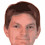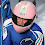## Monday, 6 August 2012

### sRGB Approximations for HLSL

The sRGB color space is non-linear. Many transformations to and from the space require the RGB components to be mapped to a linear form.

The "official" transformation for each RGB component value in the range [0,1] is:

if (C_srgb <= 0.04045)
C_lin = C_srgb / 12.92;
else
C_lin = pow((C_srgb + 0.055) / 1.055, 2.4);

This is often approximated using the "gamma 2.2" formula:

C_lin_1 = pow(C_srgb, 2.2);

This works fine, but is fairly inaccurate. The graph below uses the right-hand axis for the absolute difference:

For example, if the values are quantized to eight bits, for the sRGB component value 197/255, the linear output is 145/255 instead of 142/255. Can we do better?

In fact, if we simply change the "magic number" to 2.233333... we get better results:

C_lin_2 = pow(C_srgb, 2.233333333);

However, the "pow" functionality is either prohibitively expensive or non-existent on many platforms. So, if we limit ourselves to simple arithmetic, a good approximation I found is the cubic:

C_lin_3 = 0.012522878 * C_srgb +
0.682171111 * C_srgb * C_srgb +
0.305306011 * C_srgb * C_srgb * C_srgb;

This can be computed in HLSL using:

float3 RGB = sRGB * (sRGB * (sRGB * 0.305306011 + 0.682171111) + 0.012522878);

The reverse transformation (from linear to sRGB) is more problematic:

Again, the "official" transformation is piecewise:

if (C_lin <= 0.0031308)
C_srgb = C_lin * 12.92;
else
C_srgb = 1.055 * pow(C_lin, 1.0 / 2.4) - 0.055;

This is usually poorly approximated with the inverse of the computation of "C_lin_1":

C_srgb_1 = pow(C_lin, 0.4545454545);

In fact, the linear portion of the official graph is tiny, so an almost-perfect approximation is:

C_srgb_2 = max(1.055 * pow(C_lin, 0.416666667) - 0.055, 0);

The clamp ("max(..., 0)") is free on many platforms, but the formula does use the "pow" functionality. If we assume we only have square-root operations at our disposal, a good approximation I found was:

C_srgb_3 = 0.585122381 * sqrt(C_lin) +
0.783140355 * sqrt(sqrt(C_lin)) -
0.368262736 * sqrt(sqrt(sqrt(C_lin)));

This can be computed in HLSL using:

float3 S1 = sqrt(RGB);
float3 S2 = sqrt(S1);
float3 S3 = sqrt(S2);
float3 sRGB = 0.585122381 * S1 + 0.783140355 * S2 - 0.368262736 * S3;

An even better approximation (at the cost of an additional 'mad') is:

float3 S1 = sqrt(RGB);
float3 S2 = sqrt(S1);
float3 S3 = sqrt(S2);
float3 sRGB = 0.662002687 * S1 + 0.684122060 * S2 - 0.323583601 * S3 - 0.0225411470 * RGB;

Depending on your platform architecture, this may be faster using multiplication by a constant matrix for the final step.

1.In the "official" transformation to linear described at the top of the article, you have:

C_lin = 1.055 * pow((C_srgb + 0.055) / 1.055, 2.4);

I believe the extra 1.055 multiplier is incorrect! Shouldn't this just read:

C_lin = pow((C_srgb + 0.055) / 1.055, 2.4);

...?

1.Quite so, well spotted! Must be yet another typo: the graphs and results seem to be okay. I've corrected the code snippet. Thanks!

2.I could imagine one could have insight enough to substitute a sqrt() for pow() on some platform, but those coefficients are crazy magical! C_srgb_3 for uses 0.585122381 for example...

Do you use some curve fitting software or something that lets you investigate the effects of this stuff? How are you generating the graphs?

3.PB, the curve fitting software is actually just Microsoft Excel. The functions are fairly well-behaved (no local minima/maxima), so just using Excel's vanilla "Solver" add-in works a treat. The graphs are straight out of Excel too. I generally find it useful to blame spreadsheets for "crazy magical" results.

4.Very useful article. but value of 0.225411470 is the mistake of 0.0225411470?

5.Sigh... Well spotted! I've just looked back at my original spreadsheets and have corrected the code:

"float3 sRGB = 0.662002687 * S1 + 0.684122060 * S2 - 0.323583601 * S3 - 0.225411470 * RGB;"

near the bottom of the article has been corrected to

"float3 sRGB = 0.662002687 * S1 + 0.684122060 * S2 - 0.323583601 * S3 - 0.0225411470 * RGB;"

I've no idea what I was thinking when I copied the code to this posting, but I was obviously a bit preoccupied. Or just plain lax. As before, the graphs are correct, though.

6.Hi, Ian, really good work.

I ended just using lookup table with 16368 entries. Should easily fit in most L1 data caches on most CPUs (mine has 32K data + 32K instruction). Prefiling cache before bulk conversion, or generating lookup tables just before conversion is also good option. Good enough precision too. :)

static ubyte gamma_full(const float c) pure {
return c <= 0.0031308f
? cast(ubyte)(0.5f + 255.0f * 12.92f * c)
: cast(ubyte)(0.5f + 255.0f * (1.055f * math.pow!(float, float)(c, 1.0f / 2.4f) - 0.055f)); // Could save one mul here.
}

ubyte gamma_t;

static ubyte gamma(const float c) {
const int i = cast(int)(16383.0f * c);
return gamma_t[i & 0x3FFF];
}

Bitwise OR is to avoid clamping using conditionals on x86, and just return random color instead, without crashing.

Anyway, same results, but now it is 15 times faster than using pow + branching. And my compiler is stupid and do not inline pow too well, nor does it use sse for it. :(

7.Thanks Anonymous. That's an interesting puzzle you've got there; I hadn't really wondered about a cache-friendly conversion for CPUs until you got me thinking... So, I've had a bit of fun having a stab at this problem in the last few days. See http://chilliant.blogspot.co.uk/2015/11/srgb-integer-conversions.html

Thanks again.

8.Interesting stuff!

The example above show us how to go from Linear to sRGB, How about going from sRGB back to Linear? How can I get that data? Or other way around ehh I'm getting confused already! :- )

9.Interesting stuff!

The example above show us how to go from Linear to sRGB, How about going from sRGB back to Linear? How can I get that data? Or other way around ehh I'm getting confused already! :- )

10.Dariusz,

The sRGB to linear RGB transformation is a little bit further up the page. For clarity, here's the code again:

float3 RGB = sRGB * (sRGB * (sRGB * 0.305306011 + 0.682171111) + 0.012522878);

11.Unless I'm missing something, I get negative values for the last approximation for linear to sRGB for small linear values:

float3 sRGB = 0.662002687 * S1 + 0.684122060 * S2 - 0.323583601 * S3 - 0.0225411470 * RGB;

This occurs quite easily if you are trying to convert a small (1/255 .. 10/255) sRGB value to linear and then back using both approximations you present here.

12.This comment has been removed by the author.

13.I can't speak for its accuracy at every range, but can confirm that it does a nearly perfect job converting normals. I can't see any difference when comparing a screen shot to the original normals texture in Photoshop, which is pretty amazing. Thanks a lot for these useful tools!

14.Unreal Engine 4 / Unreal Engine 5Tuesday, 13 June 2023 at 12:17:00 UTC

for anyone coming here from Google - this method is GREAT. Here's the code for a custom material node inside the Unreal Engine :

return sRGB * (sRGB * (sRGB * 0.305306011 + 0.682171111) + 0.012522878);

and ofc you need one input called sRGB

15.This conversion is extremely lossy. Going back and forth from srgb to linear will mean extreme color distortion. Do not use, use the normal official functions instead, which are not lossy.